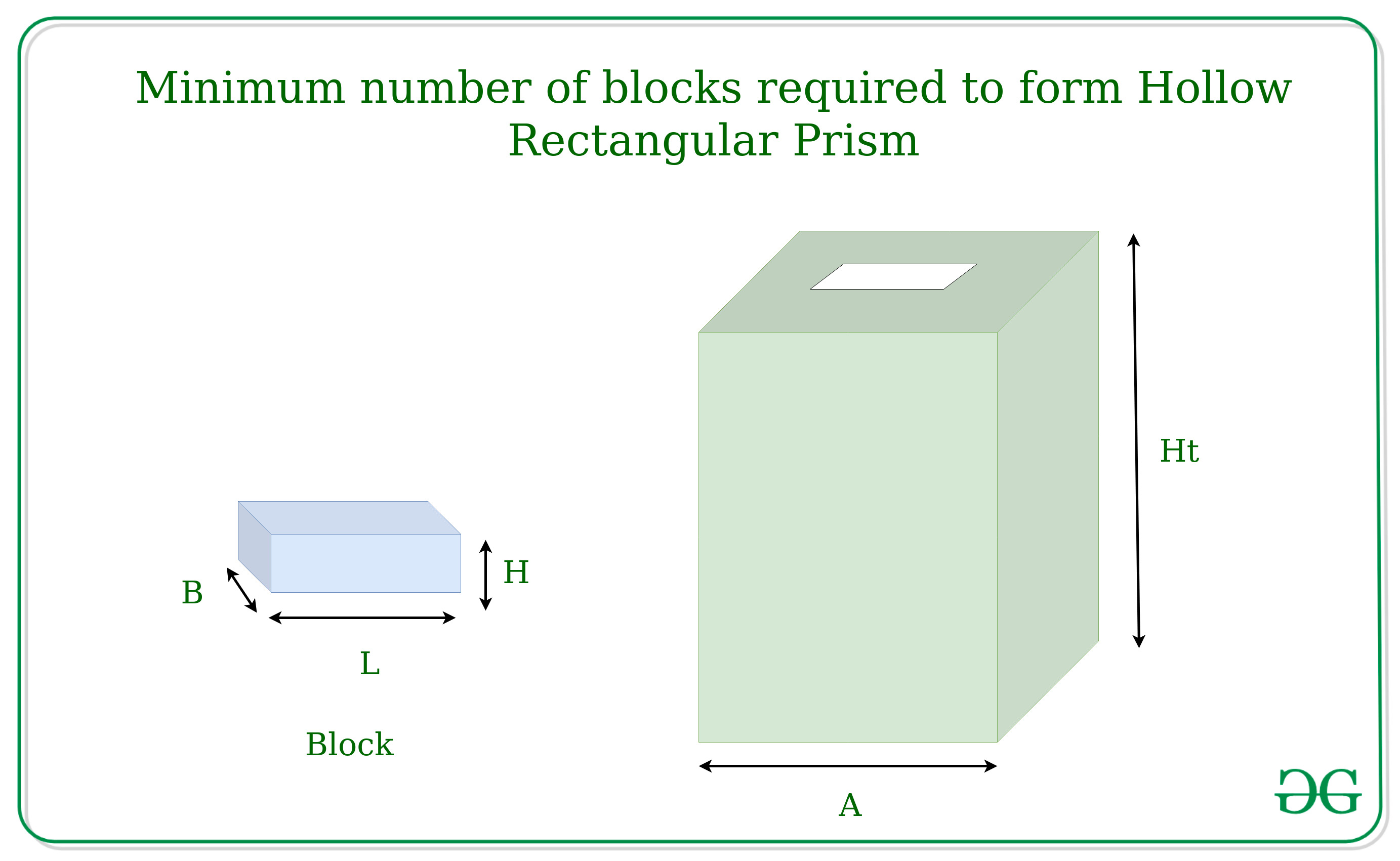Related Articles

# Minimum number of blocks required to form Hollow Rectangular Prism

• Last Updated : 18 Mar, 2021

Given the dimensions of a block L, B and H, the task is to form a Hollow Rectangular prism of length A and height Ht such that minimum no of blocks are required.
Examples:

Input: L = 40, B = 30, H = 10 & A = 500, Ht = 300
Output: 500
Input: L = 30, B = 20, H = 20, & A = 600, Ht =240
Output: 960Approach:
Calculate the minimum number of blocks required in each layer which can be done by setting the blocks in such a way that it occupies maximum length by finding the number of blocks required for the 4 sides of the rectangular prism, then choose for the block side which can be taken as the height of the block. From width or the height whichever is greater is taken as height.
Below is the implementation of the above approach:

## C++

 `// C++ Implementation to find the minimum``// no of blocks required to form``// hollow rectangular prism``#include ``using` `namespace` `std;` `// Function to display output``void` `disp(``int` `row_no, ``int` `block)``{``    ``cout << row_no * block;``}` `// Function to return minimum no of layers``// required to form the hollow prism``int` `row(``int` `ht, ``int` `h)``{``    ``return` `ht / h;``}` `// Function to calculate no of blocks``// required for each layer``void` `calculate(``int` `l, ``int` `w, ``int` `h, ``int` `a, ``int` `ht)``{``    ``// No of blocks required for each row``    ``int` `no_block = (4 * a) / l;``    ``int` `row_no;` `    ``// Check for no of layers is minimum``    ``if` `(h < w)``        ``row_no = row(ht, w);``    ``else``        ``row_no = row(ht, h);``    ``disp(row_no, no_block);``}` `// Driver function``int` `main()``{``    ``// Length, width, height of each block``    ``int` `l = 50, w = 20, h = 35;` `    ``// Side of one wall``    ``int` `a = 700;` `    ``// height of each wall``    ``int` `ht = 140;` `    ``calculate(l, w, h, a, ht);``    ``return` `0;``}`

## Java

 `// Java Implementation to find the minimum``// no of blocks required to form``// hollow rectangular prism``import` `java.util.*;` `class` `GFG{`` ` `// Function to display output``static` `void` `disp(``int` `row_no, ``int` `block)``{``    ``System.out.print(row_no * block);``}`` ` `// Function to return minimum no of layers``// required to form the hollow prism``static` `int` `row(``int` `ht, ``int` `h)``{``    ``return` `ht / h;``}`` ` `// Function to calculate no of blocks``// required for each layer``static` `void` `calculate(``int` `l, ``int` `w, ``int` `h, ``int` `a, ``int` `ht)``{``    ``// No of blocks required for each row``    ``int` `no_block = (``4` `* a) / l;``    ``int` `row_no;`` ` `    ``// Check for no of layers is minimum``    ``if` `(h < w)``        ``row_no = row(ht, w);``    ``else``        ``row_no = row(ht, h);``    ``disp(row_no, no_block);``}`` ` `// Driver function``public` `static` `void` `main(String[] args)``{``    ``// Length, width, height of each block``    ``int` `l = ``50``, w = ``20``, h = ``35``;`` ` `    ``// Side of one wall``    ``int` `a = ``700``;`` ` `    ``// height of each wall``    ``int` `ht = ``140``;`` ` `    ``calculate(l, w, h, a, ht);``}``}` `// This code is contributed by PrinciRaj1992`

## Python 3

 `# Python 3 Implementation to find the minimum``# no of blocks required to form``# hollow rectangular prism` `# Function to display output``def` `disp(row_no,block):``    ``print``(row_no ``*` `block)` `# Function to return minimum no of layers``# required to form the hollow prism``def` `row(ht, h):``    ``return` `ht ``/``/` `h` `# Function to calculate no of blocks``# required for each layer``def` `calculate(l, w, h, a, ht):``    ` `        ``# No of blocks required for each row``    ``no_block ``=` `(``4` `*` `a) ``/``/` `l``    ` `    ``# Check for no of layers is minimum``    ``if` `(h < w):``        ``row_no ``=` `row(ht, w)``    ``else``:``        ``row_no ``=` `row(ht, h)``    ``disp(row_no, no_block)` `# Driver function``if` `__name__ ``=``=` `'__main__'``:``    ``# Length, width, height of each block``    ``l ``=` `50``    ``w ``=` `20``    ``h ``=` `35` `    ``# Side of one wall``    ``a ``=` `700` `    ``# height of each wall``    ``ht ``=` `140` `    ``calculate(l, w, h, a, ht)` `# This code is contributed by Surendra_Gangwar`

## C#

 `// C# Implementation to find the minimum``// no of blocks required to form``// hollow rectangular prism``using` `System;` `class` `GFG{` `// Function to display output``static` `void` `disp(``int` `row_no, ``int` `block)``{``    ``Console.Write(row_no * block);``}` `// Function to return minimum no of layers``// required to form the hollow prism``static` `int` `row(``int` `ht, ``int` `h)``{``    ``return` `ht / h;``}` `// Function to calculate no of blocks``// required for each layer``static` `void` `calculate(``int` `l, ``int` `w, ``int` `h,``                        ``int` `a, ``int` `ht)``{``    ``// No of blocks required for each row``    ``int` `no_block = (4 * a) / l;``    ``int` `row_no;` `    ``// Check for no of layers is minimum``    ``if` `(h < w)``        ``row_no = row(ht, w);``    ``else``        ``row_no = row(ht, h);``    ``disp(row_no, no_block);``}` `// Driver function``public` `static` `void` `Main(String[] args)``{``    ``// Length, width, height of each block``    ``int` `l = 50, w = 20, h = 35;` `    ``// Side of one wall``    ``int` `a = 700;` `    ``// height of each wall``    ``int` `ht = 140;` `    ``calculate(l, w, h, a, ht);``}``}` `// This code is contributed by PrinciRaj1992`

## Javascript

 ``
Output:
`224`My Personal Notes arrow_drop_up